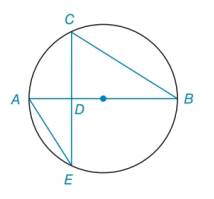Chapter 6.2, Problem 24EElementary Geometry For College St...

7th Edition
Alexander + 2 others
ISBN: 9781337614085

Solutions

Chapter
SectionElementary Geometry For College St...

7th Edition
Alexander + 2 others
ISBN: 9781337614085
Textbook Problem

In Exercises 23 to 25 , complete a paragraph proof. Given: Diameter A B ¯ ⊥ C E ¯ at D Prove: C D is the geometric mean of A D and D BTo determine

To find:

The prove,. CD is the geometric mean of AD and DB.

Explanation

Calculation:

Given, diameter AB¯CE¯ at D in the given figure.

To prove: CD is the geometric mean of AD and DB.

i.e, to prove, CD2=ADDB.

From the figure, AB¯ is the diameter and AB¯CE¯.

The diameter on a chord bisects it.

CD=DE

E and B are angles with vertex on the circle.

Thus,

mB=mAC2mE=mAC2

From the above, mB=mE

Then,

The vertical angles are equal

Still sussing out bartleby?

Check out a sample textbook solution.

See a sample solution

The Solution to Your Study Problems

Bartleby provides explanations to thousands of textbook problems written by our experts, many with advanced degrees!

Get Started

In Exercises 58, evaluate the expression. 8. |34|+|423|

Applied Calculus for the Managerial, Life, and Social Sciences: A Brief Approach

In Problems 27-32, find the general solution to each differential equation.

Mathematical Applications for the Management, Life, and Social Sciences

For j – 2k 3i – 4k 3i + j – 2k 0 (zero vector)

Study Guide for Stewart's Multivariable Calculus, 8th